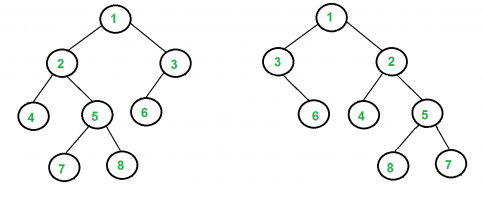The CDA Book is SHIPPING from Amazon! See here for Errata.

## Monday, August 10, 2020

### Testing Co-occurrence constraints in FHIRPath

Recently I wrote about one of the limitations of FHIRPath.  The relevant text is below:

 group.population consisting of a set of population elements, one for each criteria defined in each group. %def.group.population.all(  \$this.code ~    (%resource.group.population)[\$index].code) group.stratifier In addition, each group will contain stratifiers with a value stratum for each value defined by the stratifier criteria, for each criteria defined in the measure. %def.group.stratifier.all(  \$this.code ~     (%resource.group.stratifier)[\$index].code)and%def.group.stratifier.component.all(  \$this.code ~     (%resource.group.stratifier.stratum       .component)[\$index].code)

What was really challenging in this was a limitation of FHIRPath.  FHIRPath provides the \$this and \$index variables to define the current position within the evaluated context.  It works very much like "." does within XPath expressions.  Unfortunately, FHIR has no way to assign \$this or \$index to a variable so that it can be used with the same values in subexpressions contained inside the initial expression.

These two sets of constraints say both MORE and LESS than I actually want to say.  What they say is for each Population in %def.group.population, the code associated with population in the Measure has to match the corresponding population in the MeasureReport by numeric index.  That's a bit of BS.  Index has no semantics in the slice, code is what supplies the semantics, but there's no way to compare features of two lists that have matching semantics.

To put it simply, I'm trying to write a test that says the children of every 2 node in the structure to the left below needs to match up to with the children of the 2 node on the on the right, and the same for every other numbered node (code) that I test, without regard to the order of the codes. It's a simple graph isomorphism problem with trees (as opposed to a hard one with connected subgraphs).Given: %def.group represents groups sliced by code in Measure, and %resource.group represents groups sliced by code in MeasureReport.group, how would you match up other attribute values in def.group A to concepts in report.group B where A.code ~ B.code (these kind of constraints are called co-occurence constraints).

Take %def.group as the source of codes to work from.  The following simply won't work:

%def.group.all(
\$this.somethingElse =
%resource.group.select(code ~ \$this.code).somethingElse

The reason is that select() takes expression as an argument, meaning that inside the where(), \$this means something different than it means in %def.group.all().  To resolve this, what I'd need would be some sort of value assignment:

%def.group.all(
let that = \$this
\$this.somethingElse =
%resource.group.select(code ~ \$that.code).somethingElse
)

There are other ways to achieve a similar effect, for example, introducing a syntax that escapes to the outer level.  If \$this means the context of this expression, \$\$this could mean the context of the outer expression, and \$\$\$this could go the next level up, and I would write:

%def.group.all(
\$this.somethingElse =
%resource.group.select(code ~ \$\$this.code).somethingElse
)

However, I'm going to lose track of the difference in levels quite quickly, so I'd rather give these things a name.  I've not found any other way to accomplish this sort of test in FHIRPath.

The point of testing in this way is that I want to ensure that the graph on the right matches the graph on the left, even if it happens to have MORE stuff than is required by the graph on the left.  Because different local jurisdictions may have MORE requirements than the base requirements of a Measure needed for broader national reporting.